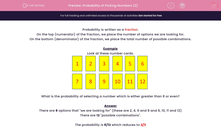# Find the Probability of Picking Numbers

In this worksheet, students will calculate the probability of choosing or not choosing certain numbers.This content is premium and exclusive to EdPlace subscribers.Key stage:  KS 2

Curriculum topic:   Maths and Numerical Reasoning

Curriculum subtopic:   Probability

Difficulty level:#### Worksheet Overview

Probability is usually written as a fraction.

On the top (numerator) of the fraction, we place the number of options we are looking for.

On the bottom (denominator) of the fraction, we place the total number of possible combinations.

Example

Look at these number cards.What is the probability of selecting a number that is either even or greater than 8?

There are 8 options that we are looking for (these are 2, 4, 6 and 8 and 9, 10, 11 and 12)

There are 12 possible combinations.

The probability is 8/12 which reduces to 2/3

Let's have a go at the questions now.

### What is EdPlace?

We're your National Curriculum aligned online education content provider helping each child succeed in English, maths and science from year 1 to GCSE. With an EdPlace account you’ll be able to track and measure progress, helping each child achieve their best. We build confidence and attainment by personalising each child’s learning at a level that suits them.

Get started